# How imshowpair and imfuse work

In this post, I'll explore how imshowpair and imfuse work.
Reason: I was curious.
Last month, I wrote about registering several hand-held photographs together. In that post, I used imshowpair several times.
Here are two of the images:
imshow('A.jpg')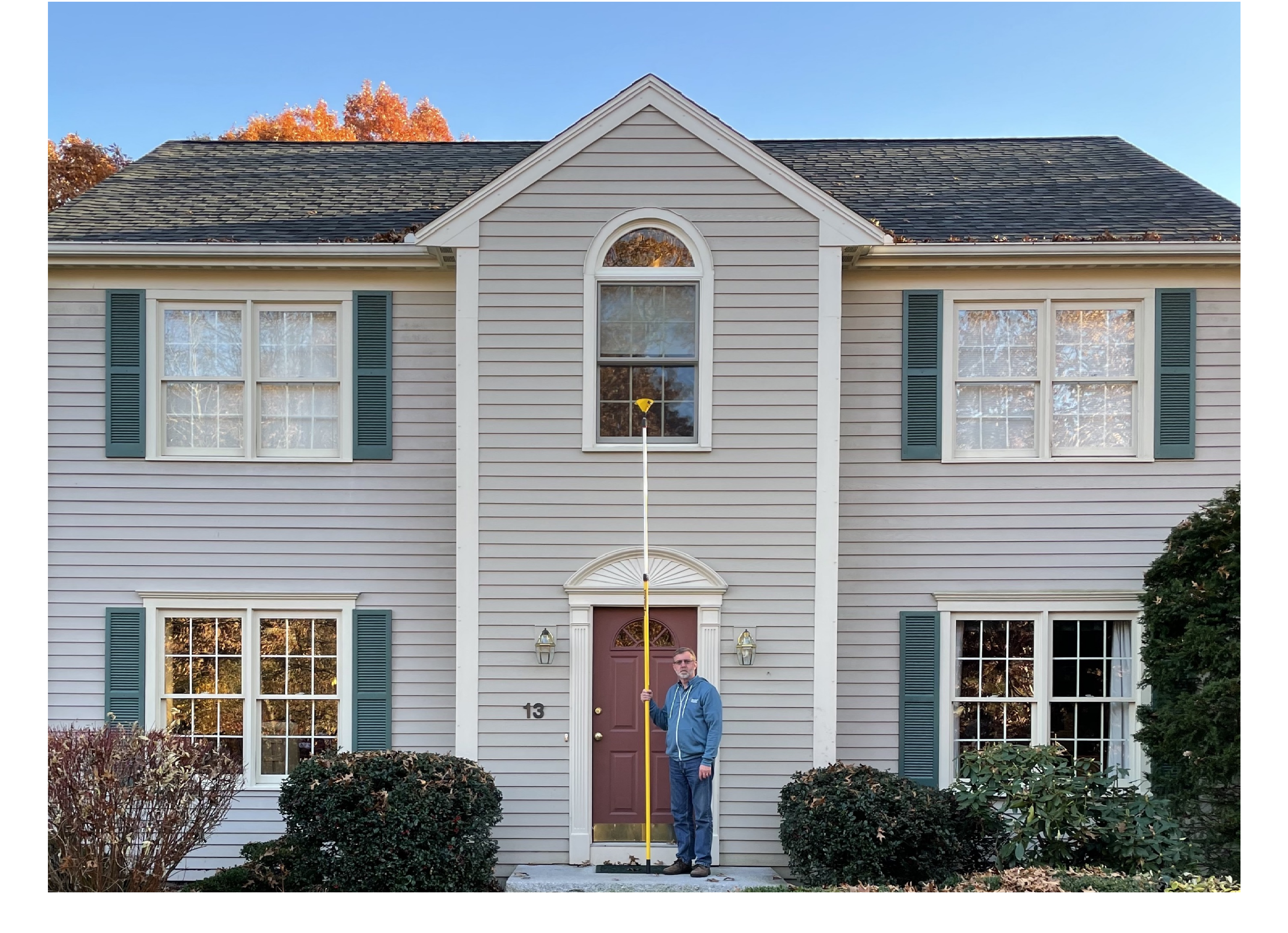imshow(B)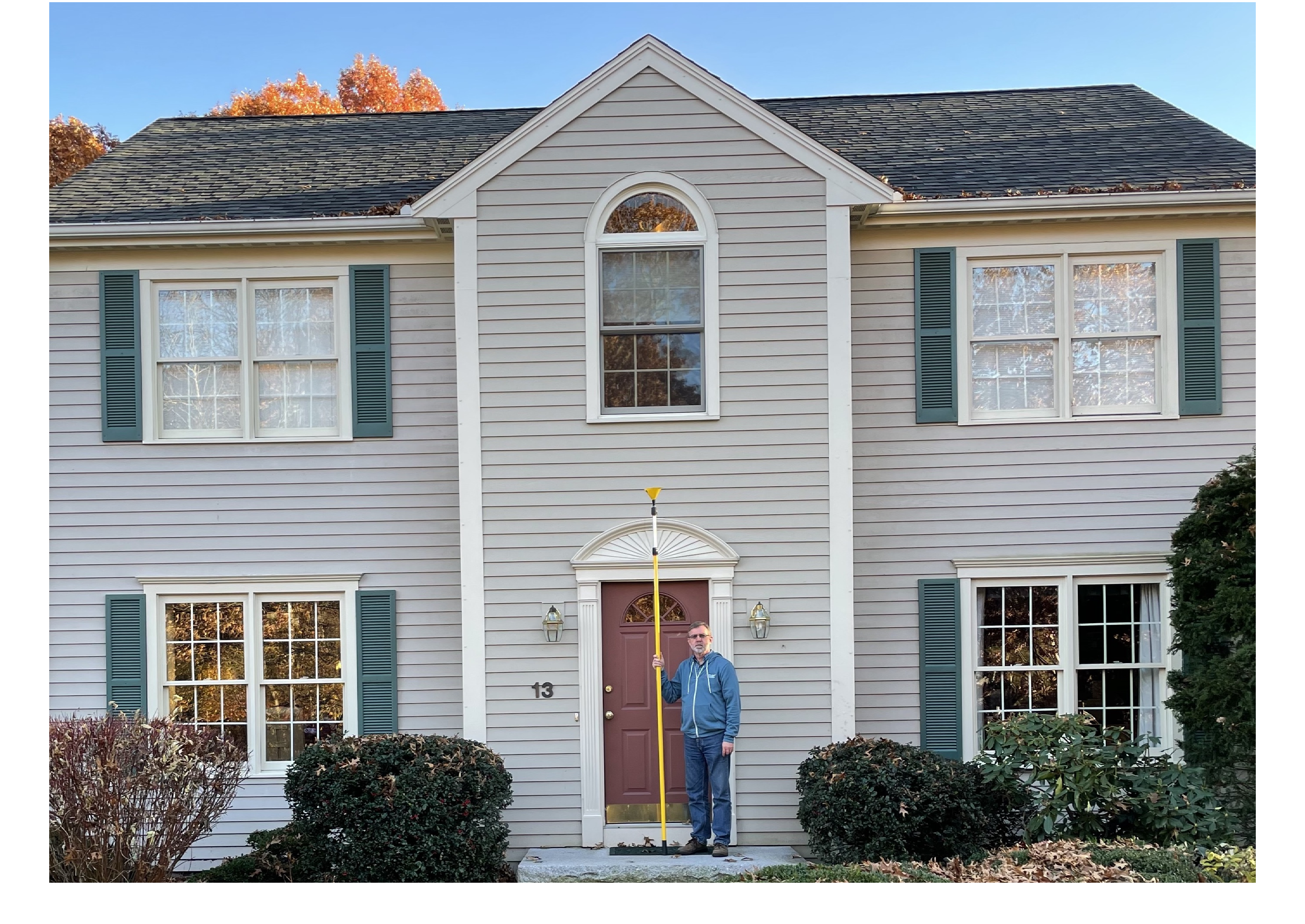And here is imshowpair in use:
imshowpair(A,B)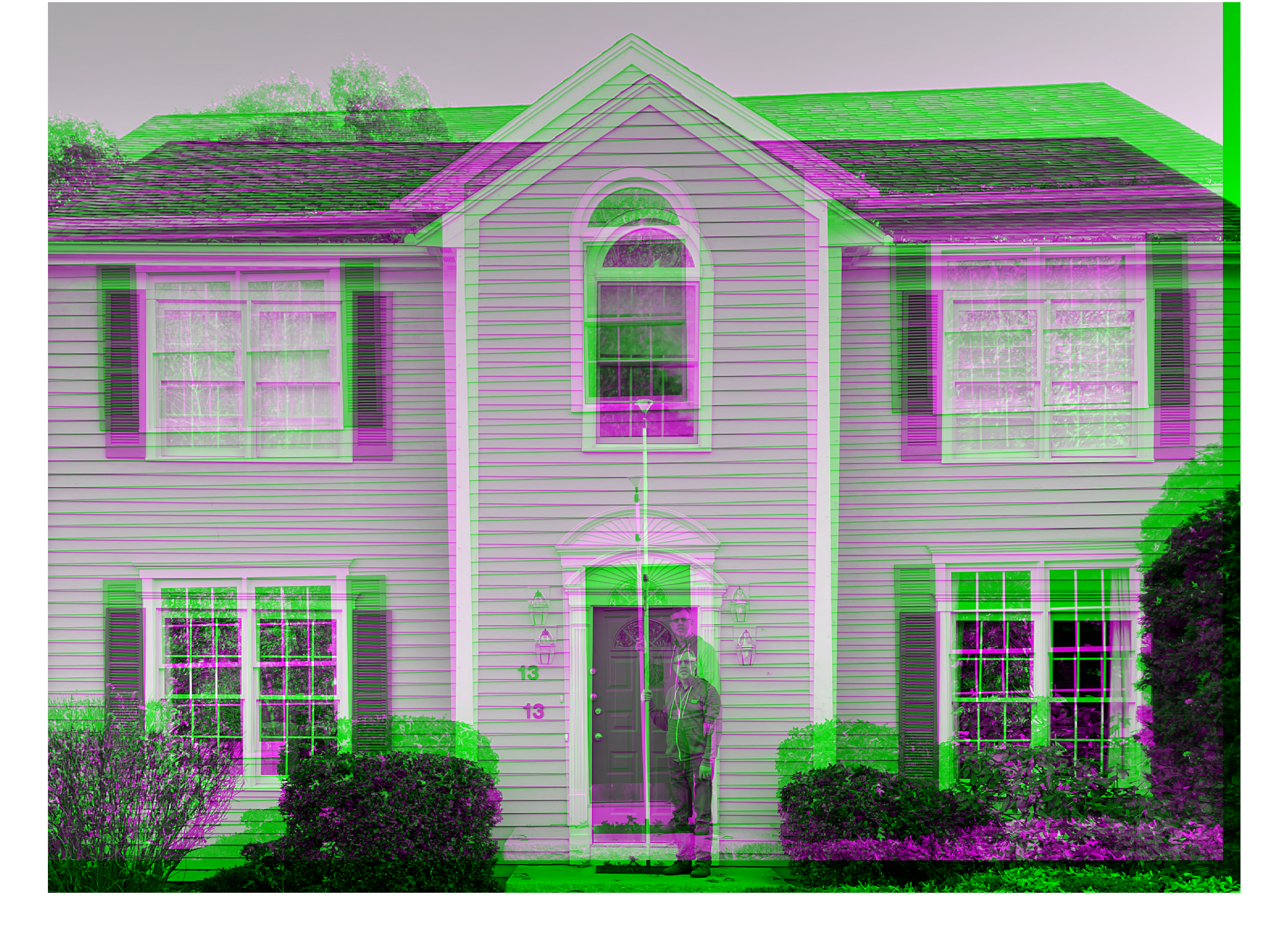I wanted to know how this function works. Today, I thought I would share with you what I found and how I found it.
Many functions in Image Processing Toolbox ship as MATLAB source code. When this is the case, I always start my code exploration by using the Debugger to follow the code as it executes, a step at a time. Here is the beginning of my code dive: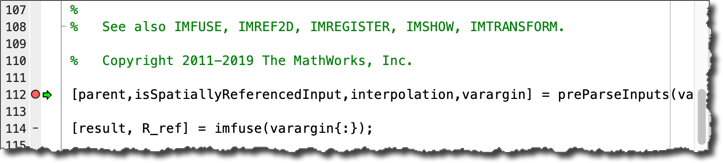The first thing I notice is that the parsed input arguments are all passed along immediately to imfuse. A few lines later, the results from imfuse are displayed using imshow.
Aha! The function imfuse must be really how this is all computed. So, I continue to follow execution in the Debugger by stepping into imfuse: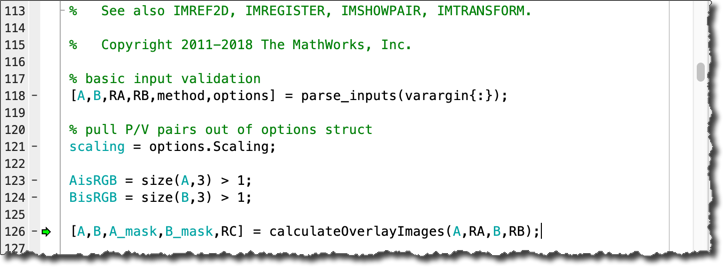The first interesting-looking step is calculateOverlayImages. (Note: the variables RA and RB are the spatial references that define where each image lies in space. Since I didn't provide this information as input arguments, these simply set up the default spatial references, where the horizontal and vertical coordinates are the same as the pixel subscripts.) This is a local function inside of imfuse.m. From looking over its code, I can see that it does the following:
1. Finds an output grid in space that is big enough to cover the location of image A as well as image B.
2. Sets each pixel size (width and height) in the output grid to be the smallest of the corresponding pixel sizes in A and B.
3. Resamples each image into that output grid.
4. Computes two mask images that correspond to the location of images A and B within the output grid.
Continuing to follow the code execution, I see that the next interesting point is the call to the local function local_createRGB. Here is that function: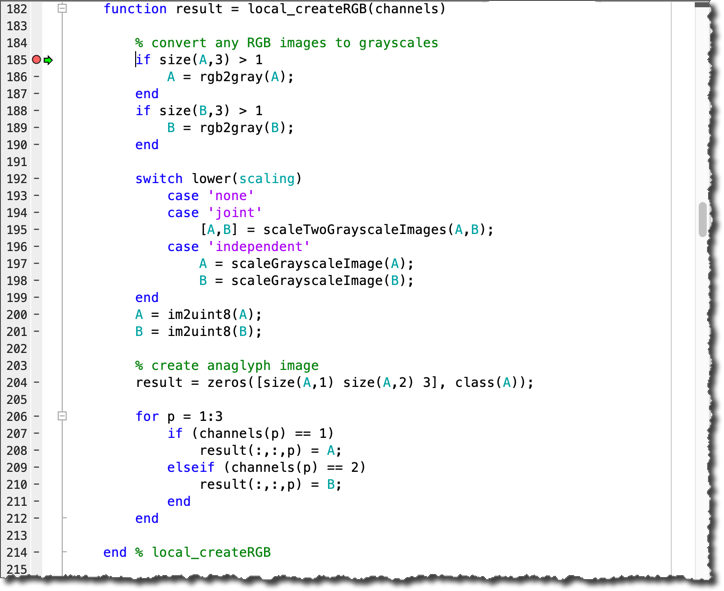The two images are both converted to grayscale (if necessary), and then each image is scaled. The independent scaling method, which I also peeked at, simply scales each image to take up the full black-to-white dynamic range. Then, the images are converted to the uint8 data type using the appropriate type-specific scaling as provided by im2uint8.
Finally, the now-grayscale images A and B are inserted directly into the red, green, and blue channels of the output image. By default, image A is inserted into the green channel, and image B is inserted into the red and blue channels.
The function imshowpair is convenient when you just want to display the fused result, but you don't need the result to save or for further computation. If you need the fused result, then call imfuse directly.
The functions imshowpair and imfuse support other fusion methods as well. I'll leave you with the results of the 'checkerboard' method.
imshowpair(A,B,'checkerboard')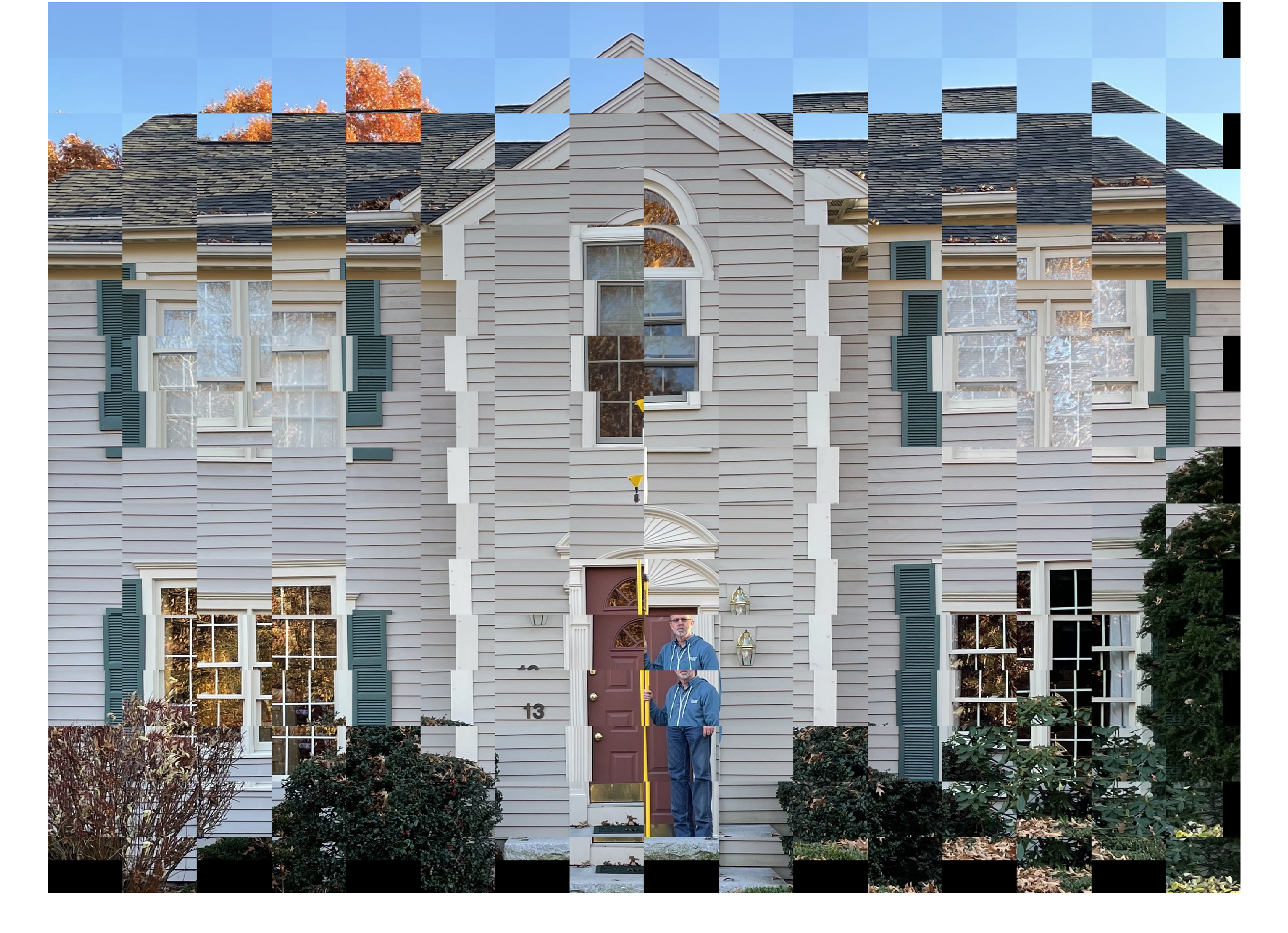|